1800-1023-196

+91-120-4616500

# Nuclear Stability

## Nuclear Stability

Nuclides can be grouped on the basis of nuclear stability, i.e. stable and unstable nucleus. The most acceptable theory about the atomic nuclear stability is based upon the fact that the observed atomic mass of all known isotopes (except hydrogen) is always less from the sum of the weights of protons and neutrons present in it. Electron (b- particle) from a radioactive nucleus may be regarded as derived from a neutron in the following way,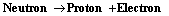Similarly, photons are produced from internal stresses within the nucleus.

The stability of nucleus may be discussed in terms of any one of the following,

Nuclear Binding Energy and Mass defect : It is observed that atomic mass of all nuclei (except hydrogen) is different from the sum of masses of protons and neutrons. The difference is termed mass defect.

Mass defect = Total mass of nucleons – obs. atomic mass

The mass defect is converted into energy. This energy is called the binding energy. This is the energy required to break the nucleus into is constituents (p and n).

Binding energy = Mass defect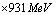The stability of the nucleus is explained on the value of binding energy per nucleon and not on the basis of total binding energy . Binding energy per nucleon is maximum (8.7 MeV) in the case of iron (56). The value of binding energy per nucleon can be increased either by fusion of lighter nuclei or fission of heavier nuclei.

Value of binding energy predicts the relative stability of the different isotopes of an element. If the value of binding energy is negative, the product nucleus or nuclei will be less stable than the reactant nucleus. Thus the relative stability of the different isotopes of an element can be predicted by the values of binding energy for each successive addition of one neutron to the nucleus.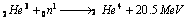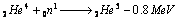Therefore,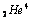is more stable than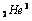and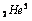.

## NEET & AIIMS Exam Sample Papers

 AIIMS SAMPLE PAPERS View More NEET SAMPLE PAPERS View More